# Editing and Copying Formulas in Microsoft Excel

#### Editing a Formula

To edit a formula in Excel, use the following procedure. The following example in this Excel formula tutorial uses an incorrect cell reference.

1. Select the cell with the formula you want to correct to make it active.
2. Select the Formula Bar. Excel highlights the cell references in the current formula.

For more on instructor-led Microsoft Excel classes in Los Angeles call us on 888.815.0604.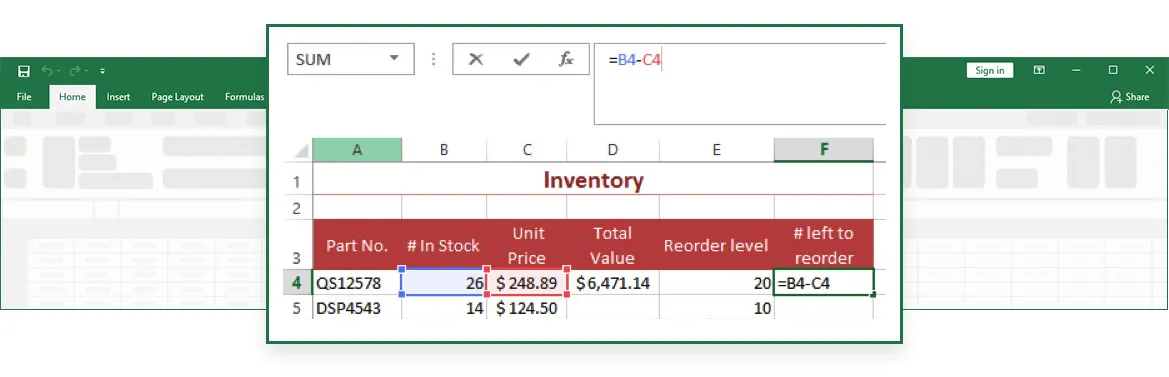Highlight the operator or cell references and either type over with the correct reference or operator, or select the correct cell to replace a cell reference.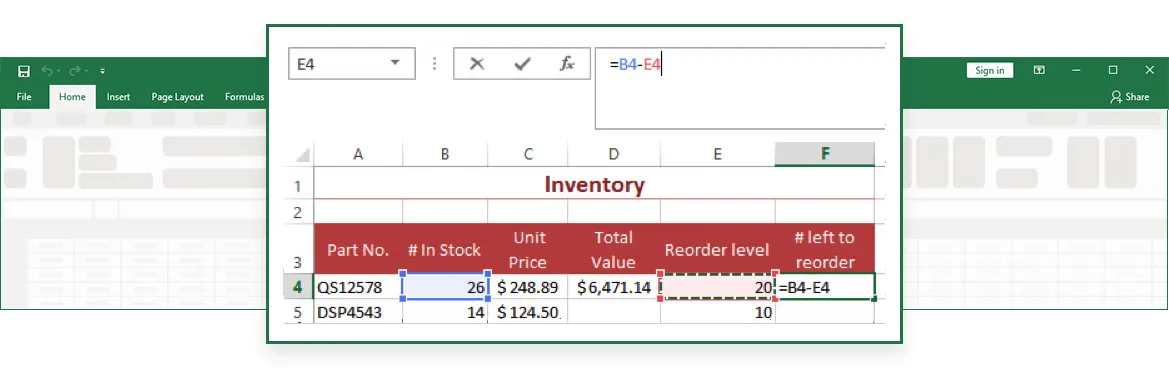Press ENTER to complete the formula. Excel calculates the formula and moves to the next row.

#### Copying a Formula

To copy and paste a formula in Excel, use the following procedure.

1. Select the cell with the formula you want to copy. You can also click on the cell and use the keyboard shortcut: CTRL + C.
2. Select Copy from the Home tab on the Ribbon.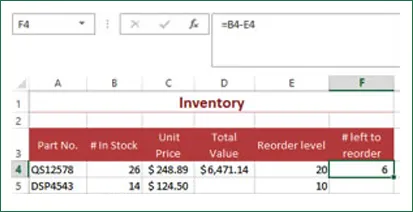Excel highlights the cell whose contents you are copying. This will remain highlighted until you finish pasting, in case you want to paste the cell contents more than once.

3.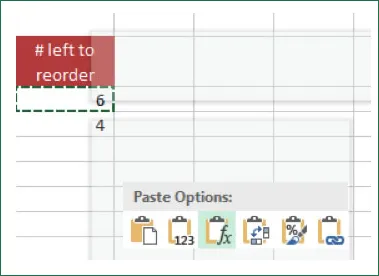Select the cell where you want to copy the formula. Excel displays a number of paste options. To paste a formula, select Paste or Paste formula. Note that as you hover your mouse over the paste options, the rest of the context menu is dimmed. You can also select the cell and use the keyboard shortcut: CTRL + V.

4. Press ENTER to complete the formula. Excel calculates the formula and moves to the next row.

#### Copying a formula with an Absolute Reference

To copy a formula in Excel with an absolute reference, use the following procedure.

1. Create a new column labeled Taxes.
2. Select the Taxes column for the first product (cell E4).
3. Enter the = sign to begin the formula.
4. Select cell B16 to use it as the first value in the formula. Excel enters the reference as part of the formula. Use the Formula Bar to enter dollar signs before the column and the row (i.e., \$B\$16).
5. Enter * and the relative reference in the Total Value column.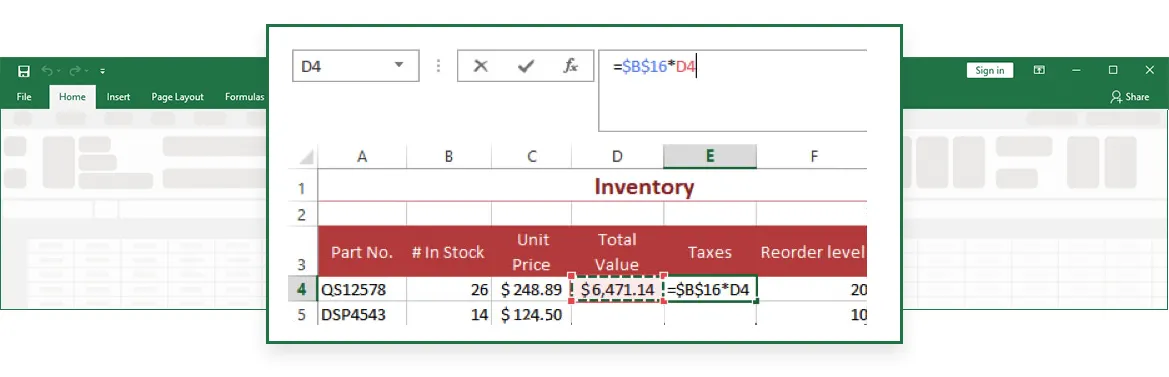1. Press ENTER to complete the formula. Excel moves to the next row and performs the calculations in the formula.

#### Group Excel training

Through our network of local trainers we deliver onsite group Microsoft Excel training right across the country. View our Excel training testimonials or obtain a quote for a customized Excel class.